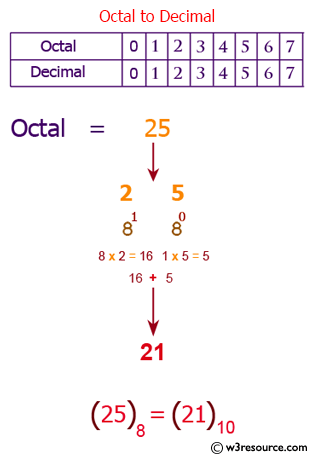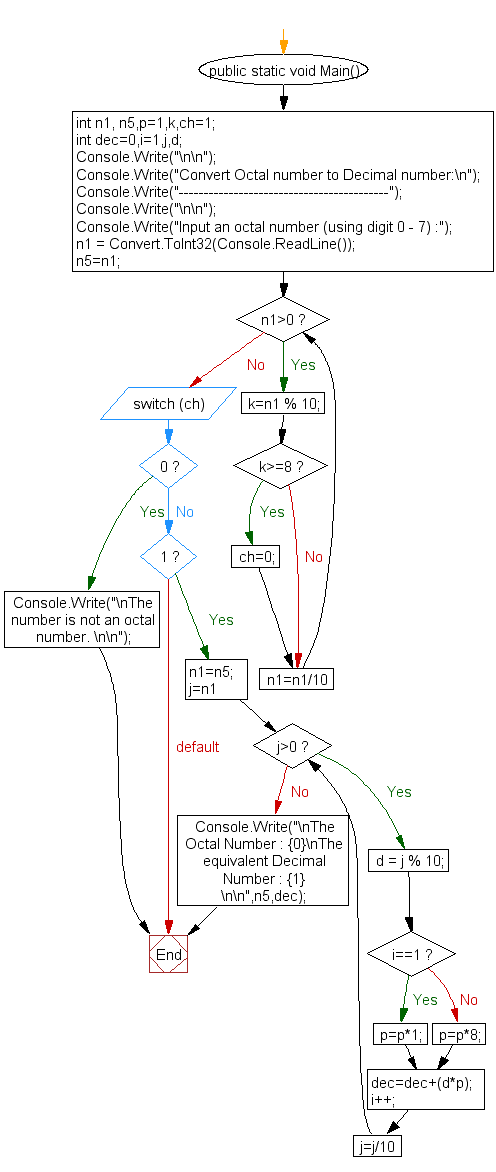﻿ C# - Convert Octal number to Decimal number - w3resource# C# Sharp Exercises: Convert Octal number to Decimal number

## C# Sharp For Loop: Exercise-51 with Solution

Write a program in C# Sharp to convert a Octal number to Decimal number without using an array, function and while loop.

Pictorial Presentation:Sample Solution:

C# Sharp Code:

``````
using System;
public class Exercise51
{
public static void Main()
{       int n1, n5,p=1,k,ch=1;
int dec=0,i=1,j,d;

Console.Write("\n\n");
Console.Write("Convert Octal number to Decimal number:\n");
Console.Write("------------------------------------------");
Console.Write("\n\n");

Console.Write("Input an octal number (using digit 0 - 7) :");
n5=n1;

for(;n1>0;n1=n1/10)
{
k=n1 % 10;
if(k>=8)
{
ch=0;
}
}

switch(ch)
{
case 0 :
Console.Write("\nThe number is not an octal number. \n\n");
break;
case 1:
n1=n5;
for (j=n1;j>0;j=j/10)
{
d = j % 10;
if(i==1)
p=p*1;
else
p=p*8;

dec=dec+(d*p);
i++;
}
Console.Write("\nThe Octal Number : {0}\nThe equivalent Decimal  Number : {1} \n\n",n5,dec);
break;
}
}
}
```
```

Sample Output:

```Convert Octal number to Decimal number:
------------------------------------------
Input an octal number (using digit 0 - 7) :5
The Octal Number : 5
The equivalent Decimal  Number : 5
```

Flowchart:C# Sharp Code Editor:

Contribute your code and comments through Disqus.

What is the difficulty level of this exercise?

Test your Programming skills with w3resource's quiz.

﻿